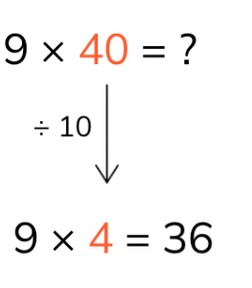Multiplying multiples of 10 using the zero rule

# Multiplying multiples of 10 using the zero rule

Students learn to multiply using the zero rule using tens.8,000 schools use Gynzy92,000 teachers use Gynzy1,600,000 students use Gynzy

## General

The students learn to multiply multiples of 10 using the zero rule.

## Standards

CCSS.Math.Content.3.NBT.A.3

## Learning objective

Students will be able to multiply multiples of 10 using the zero rule.

## Introduction

The students make a bingo card, on which they fill in answers from the 7, 8, and 9 times tables. You state a multiplication problem from one of these times tables and the students check whether the answer of this problem is on their bingo card. If it is, they may cross out the number. Continue until one student has bingo.

## Instruction

First explain that a times table problem is a multiplication problem in which you determine how many times of something you have. For example, in the 10 times table you have 10 different numbers of times. Explain the zero rule with help from the problem 9 x 40. Show how you solve the problem using a helping problem. The helping problem is the problem, for which a 0 is taken away from the tens number. Then the helping problem can be solved. To solve the original problem, add a 0 to the answer of the helping problem.
For example: 9 × 40 = ? Then the helping problem is 9 × 4 = 36. Now a 0 is added back to the answer. So the 36 becomes 360. This is the answer to the problem. Discuss the examples of problems with helping problems. Explain that this is how you can multiply in steps with one tens number using the zero rule. Check whether the students can make the helping problems for the multiplication problems given. Then check if they can solve the problems using the zero rule. Show the table and explain to the students how they should read it. This is how you multiply the number in the row with the number in the column. You put the answer into the table. Check whether the students can solve the problems in the table.

Check whether the students can multiply multiples of 10 using the zero rule with the following questions:
- What is a helping problem?
- How do you multiply using the zero rule?
- How do you solve 5 × 40?

## Quiz

The students test their understanding of multiplying multiples of tens with the zero rule through ten exercises. For some they must solve helping problems and multiplication problems using the zero rule. Other exercises they must fill in a multiplication table.

## Closing

Discuss once again with the students that it is important to be able to multiply with a tens number with the zero rule, so that you can calculate how much you have in a multiplication problem with one tens number. Ask how they can solve table problems in steps. Check with the problems if students can apply what they have learned. As a closing activity the students play memory, for which they must make pairs with a problem and its helping problem. For example: 6 x 7 and 60 x 7. Have students move two cards to the side. If they have a pair, they can go again. Also ask if they know the answer to the problem. They get extra points if they do.

## Teaching tips

Have students that have difficulty with multiplying with a tens number with the zero rule first practice with the 1 to 10 times tables. For this students can use concrete materials, such as blocks, if they need them or have the students draw out the multiplication problems on a piece of scrap paper. If students have difficulty with multiplication, you can have them practice with adding in jumps of 10.

## Instruction materials

Paper for bingo and blocks

### The online teaching platform for interactive whiteboards and displays in schools

• Save time building lessons

• Manage the classroom more efficiently

• Increase student engagement